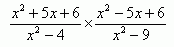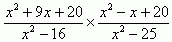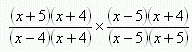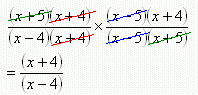SEARCH HOMEMath Central Quandaries & QueriesQuestion from sergio, a student: how to simply (x^2 +5x+6)/(x^2 - 4) x (x^2 -5x+6)/(x^2-9)Hi Sergio.

You sent us this:which is a lot like this example I am going to solve:To simplify these kinds of rational algebraic expressions, we need to factor things as much as possible.The denominators were easy to factor because each is a difference of squares. The numerators I had to try combinations of the factors of 20 to solve. Since we are multiplying two fractions, we can treat it as one big fraction and just cancel matching factors on the top and bottom.So now I've simplified my example problem. Solve yours just the same way, Sergio.

Cheers,
Stephen La Rocque.Math Central is supported by the University of Regina and The Pacific Institute for the Mathematical Sciences.